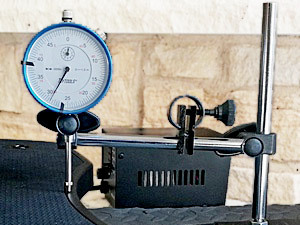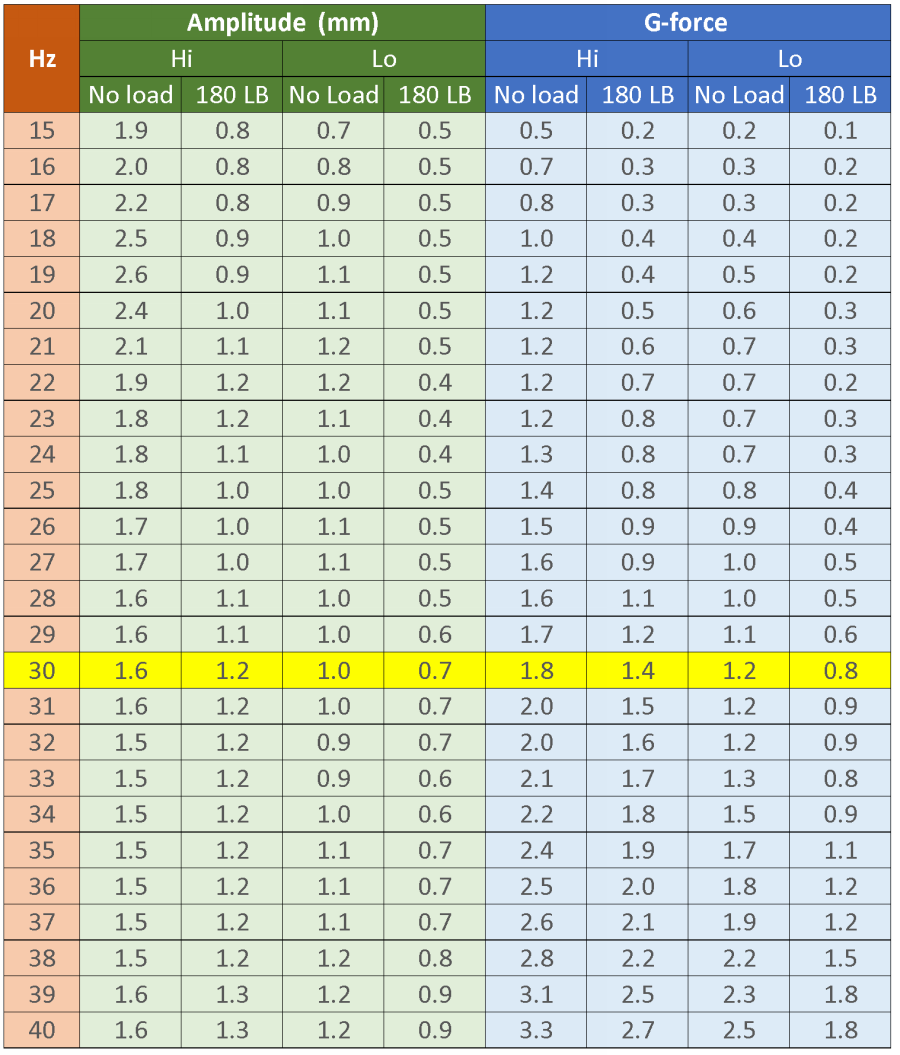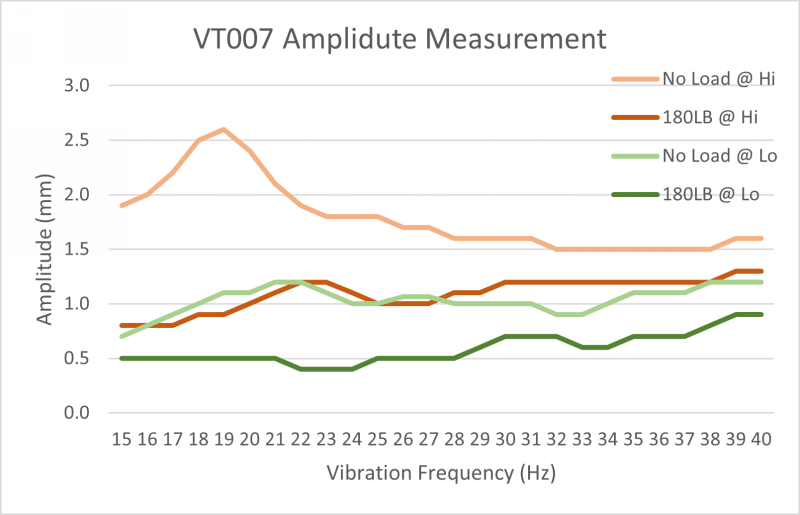devoted to
making better vibration platesJphoto

# VT007 Amplitude & G-force SheetWe conducted amplitude measurement on two randomly selected VT007 machine. The horizontal and vertical displacement of the upper plate during vibration is measured under the following variables:

1. Amplitude settings: Hi and Lo
2. Vibration Frequency: 15Hz - 40Hz

Vibration G-force are calculated based on these measurement results.

## VT007 Amplitude Measurement Report

VT007 Vertical Amplitude Measurement & G‑force Calculation## VT003F Amplitude Chart* Amplitude in the chart is the peak-to-peak amplitude

## Test Result Highlights

 Max Amplitude on No Load 3.05  mm at 20 Hz Max Amplitude on 180LB 1.47 mm at 40 Hz Max G-Force on No Load 2.86 at 40 Hz Max G-Force on 180LB 2.37 at 40Hz

## Variables Affecting Amplitude

#### Weight of eccentric wheels

For a linear vibration plate, the vibration is generated by the combination of a set of eccentric wheels and a set of springs. The eccentric wheels, when rotated, create cycling centrifugal force that moves the upper plate to move in a circle. The heavier the eccentric wheels or the higher the rotation speed, the larger the centrifugal force. The springs regulate the movement by allowing more movement on vertical direction and less on the horizontal direction. The displacement of the spring, which becomes the amplitude of the vibration plate, is in a linear relationship with the centrifugal force. That is why this type of vibration is called linear vibration.

#### Frequency Response

Larger centrifugal force may not necessarily results in higher vibration amplitude. As a forced vibration system, vibration plate has a frequency response pattern, which is the pattern of vibration amplitude in relation to the input vibration frequency. The frequency response pattern is complicated. The highest amplitude usually happens when the input frequency equals the natural frequency of the object, which is called resonance. Compared with VT003F, VT007 has better frequency response at higher frequency. Therefore VT007 achieves higher G-force.

Linear vibration plate is a flexible link structure. The vibration amplitude yields to the force that presses the sporting springs. The pressing force is from the load - the body weight. The load and the amplitude are in a linear relationship: the larger the load, the lower the amplitude.

VT007's two amplitude settings, Hi and Lo, are made available by switching the weight of the eccentric wheel. At Hi, 3 pair of eccentric wheels are engaged, and at Lo, one pair of eccentric wheels are engaged.

#### Amplitude Calculation

In our test, we only measured the amplitude for no load and 180LB load (body weight). We can user formula to calculate amplitude for other body weight

In linear vibration, theoretically the amplitude is in inverse proportion to the body weight. Therefore when the amplitudes at 0 weight and 180Lb weight are known, we can work out the formula to calculate the amplitude for different body weight. See below.

Amplitude Setting: Low

 Frequency Amplitude Formula (mm) 15Hz Amplitude = 0.56 + 0.0017 * Weight 20Hz Amplitude = 0.51 + 0.0039 * Weight 25Hz Amplitude = 0.36 + 0.0039 * Weight 30Hz Amplitude = 0.76 + 0.0008 * Weight 35Hz Amplitude = 0.86 40Hz Amplitude = 1.07

Amplitude Setting: High

 Frequency Amplitude Formula (mm) 15Hz Amplitude = 0.76 + 0.0079 * Weight 20Hz Amplitude = 0.76 + 0.0127 * Weight 25Hz Amplitude = 0.76 + 0.0059 * Weight 30Hz Amplitude = 1.2 + 0.0017 * Weight 35Hz Amplitude = 1.17 + 0.0014 * Weight 40Hz Amplitude = 1.47 + 0.0017 * Weight

When you have the amplitude and frequency, we can calculate out the G-force.